Vectors and conics

This free course is available to start right now. Review the full course description and key learning outcomes and create an account and enrol if you want a free statement of participation.

Free course

2 Vectors

2.1 Definitions

In this section we introduce an alternative way of describing points in the plane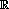2 or in three-dimensional space3; namely, by using vectors.

You meet vectors such as velocity and acceleration in everyday life; these have in common the fact that to specify each fully, we must give both its size and also its direction. For example, if you are driving along a road, then you are interested both in how fast your car is moving (that is, its speed) and also in what direction. Physical quantities that are uniquely determined only if we know both their size and their direction are called vectors. We shall use the term magnitude for the size of the physical quantity represented.

For example, a velocity of 50 km h−1 north-east differs from a velocity of 90 km h−1 north-east, and both of these differ from a velocity of 50 km h−1 south-west.

By contrast, some physical quantities (such as temperature and volume) have only a magnitude – they have no direction associated with them. We call such quantities scalars.

Definitions

A vector is a quantity that is determined by its magnitude and direction.

A scalar is a quantity that is determined by its magnitude.

We can represent a vector geometrically by a line segment in2 or in3. The length of the line segment is a measure of the magnitude of the vector, and the direction of the line is the same as the direction of the vector; we indicate the direction of the line with an arrow, as shown below. A vector represented by a line segment from A to B is often written as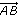; the magnitude of the vector is proportional to the distance between A and B, and the arrow indicates the direction of the vector.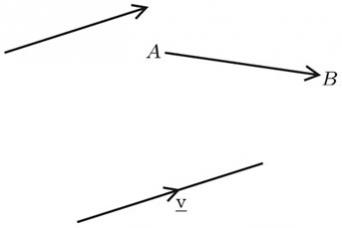Often we use single letters, such as a, b, p, q or v, to denote vectors. Vectors are usually distinguished in print by the use of a bold typeface, or in hand-written work by underlining the letters (for example, v). This is a useful convention, as it reminds us that they are vector quantities.

We use two pairs of vertical lines to denote the magnitude of a vector; thus we denote the length of a vector v by the symbol ‖v‖.

There is just one vector which does not fit conveniently into the above definition; namely, the zero vector. This arises quite naturally, however. For, just as we can imagine a car travelling at 50 km/h NE (we abbreviate north-east to NE, and so on), it makes sense to say that a car is stationary; in this case, the magnitude of the velocity of the car is zero, but we can assign any direction that we please to its velocity.

Definition

The zero vector is the vector whose magnitude is zero, and whose direction is arbitrary. It is denoted by the symbol 0.

In order to gain a feeling for how we should build up an arithmetic for vectors that agrees with our intuition, we shall now look at some geometric examples of vectors in the plane.

For convenience, we shall use compass bearings to describe the directions of the vectors.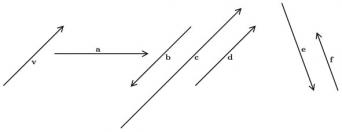In the diagram above, v is the vector 3 cm NE. This vector is different from the vectors a, b, e and f as its direction differs from their directions. Also, v is a different vector from c although it has the same direction as c, since its magnitude is different from that of c. However, v and d are the same vector, since they have the same magnitude and direction. Note that the geometric representations of equal vectors do not need to start at the same point and finish at the same point. They need only be the same length and in the same direction.

Definition

Two vectors a and b are equal if

they have the same magnitude (‖a‖ = ‖b‖)

and

they are in the same direction.

We write a = b.

Now, although the vectors v and b are unequal, they are closely related: they have the same magnitude (3 cm), and their directions are exactly the opposite of each other (NE and SW). We say that b is ‘minus v’, and write this as b = −v. If we write v in terms of a line segment AB in the form, then −v can be expressed as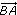.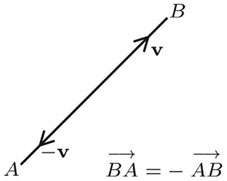Definition

The negative of a vector v is the vector with the same magnitude as v, but the opposite direction. It is denoted by −v.

M208_1